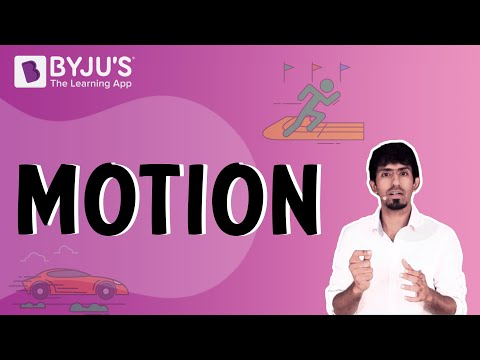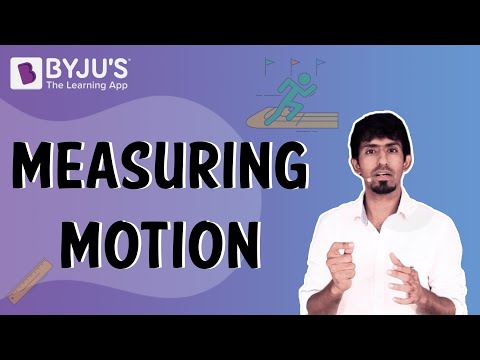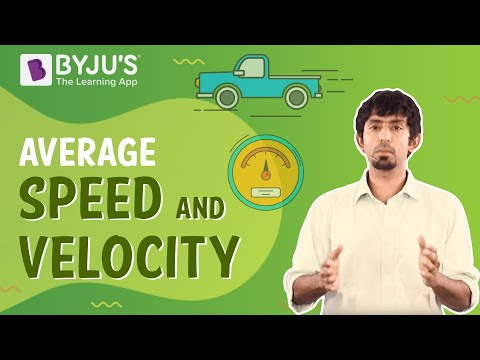# Motion Class 9 CBSE Notes - Chapter 8

## Understanding Motion

### Reference point and reference frame

• To describe the position of an object we need a reference point or origin. An object may seem to be moving to one observer and stationary to another.
• Example: A passenger inside a bus sees the other passengers to be at rest, whereas an observer outside the bus sees the passengers to be in motion.
• In order to make observations easy, a convention or a common reference point or frame is needed. All objects must be in the same reference frame.To know more about frame of reference, visit here.

## Distance and Displacement

The magnitude of the length covered by a moving object is called distance. It has no direction.

Displacement is the shortest distance between two points or the distance between the starting and final positions with respect to time. It has magnitude as well direction.

Displacement can be zero, but distance cannot.

To know more about Distance and Displacement, visit here.

### Magnitude

Magnitude is the size or extent of a physical quantity. In physics, we have scalar and vector quantities.

Scalar quantities are only expressed as magnitude. E.g: time, distance, mass, temperature, area, volume

Vector quantities are expressed in magnitude as well as the direction of the object. E.g: Velocity, displacement, weight, momentum, force, acceleration, etc.

## Time, Average Speed and Velocity

### Time and speed

Time is the duration of an event that is expressed in seconds. Most physical phenomena occur with respect to time. It is a scalar quantity.

Speed is the rate of change of distance. If a body covers a certain distance in a certain amount of time, its speed is given by

$Speed =\frac{Distance}{Time}$

Average speed = Total distance travelled / Total time taken

### Uniform motion and non-uniform motion

When an object covers equal distances in equal intervals of time it is in uniform motion.

When an object covers unequal distances in equal intervals of time it is said to be in non-uniform motion.

To know more about Uniform Motion and Non-Uniform Motion, visit here.

### Velocity

The Rate of change of displacement is velocity. It is a vector quantity. Here the direction of motion is specified.

$Velocity = \frac{Displacement}{Time}$

Average velocity = (Initial Velocity + Final velocity)/2 = $\frac{ u+ v}{2}$.

#### For More Information On Average Speed and Velocity, Watch The Below Video:To know more about Average Speed and Average Velocity, visit here.

## Acceleration

The rate of change of velocity is called acceleration. It is a vector quantity. In non-uniform motion, velocity varies with time, i.e., change in velocity is not 0. It is denoted by “a”

Acceleration Change in Velocity / Time

(OR)

$a = \frac{v-u}{t}$

Where, t (time taken), v (final velocity) and u (initial velocity).

To know more about Acceleration, visit here.

## Motion Visualised

### Distance-Time graph

• Distance-Time graphs show the change in position of an object with respect to time.
• Linear variation = uniform motion and non-linear variations imply non- uniform motion
• The slope gives us speed
• OA implies uniform motion with constant speed as the slope is constant
• AB implies the body is at rest as the slope is zero
• B to C is non-uniform motion

To know more about Distance-Time Graph, visit here.

### Velocity-Time Graph

• Velocity-Time graphs show the change in velocity with respect to time.
• Slope gives acceleration
• The area under the curve gives displacement
• Line parallel to x-axis implies constant velocity-

OA = constant acceleration, AB = constant velocity, BC = constant retardation

To know more about Velocity-Time Graph, visit here.

## Equations of Motion

The motion of an object moving at uniform acceleration can be described with the help of three equations, namely

(i) v = u + at

(ii) v2 – u2 = 2as

(iii) s = ut + (1/2)at2

To know more about Equations of Motion, visit here.

### Derivation of velocity-time relation by graphical method

A body starts with some initial non-zero velocity at A and goes to B  with constant acceleration a.

From the graph BD = v (final velocity) – DC = u (initial velocity)…………..(eq 1).

BD = BC – DC……………..(eq 2).

We know acceleration a = slope = $\frac{BD}{AD}$ or AD = OC = t (time taken to reach point B).

Therefore  BD = at………………….(eq 3).

Substitute everything  we get : at = v – u.

Rearrange to get v = u + at.

### Derivation of position-time relation by graphical method

A body starts with some initial non-zero velocity at A and goes to B  with constant acceleration a

Area under the graph gives Displacement =A(ΔABD)+A(OADC)=($\frac{1}{2}$AD×BD)+OA×OC ……………(eq 1)

OA = u , OC = t and BD = at

Substituting in (eq 1) we get s= $ut + \frac{1}{2}at^{2}$

### Derivation of position-velocity relation by graphical method

A body starts with some initial non-zero velocity at A and goes to B  with constant acceleration a

Displacement covered will be the area under the curve which is the trapezium OABC.

We know the area of trapezium is s= $\frac{(OA+BC)}{2*OC}$

OA = u and BC = v and OC = t

Therefor, s=  $\frac{(v+u)}{2*t }$ ……………(eq 1)

We also know that  t =$\frac{(v-u)}{a}$ ……………..(eq 2)

Substitute (eq 2) in (eq 1) and arrange to get

v2−u2=2as

## Uniform Circular Motion

### Uniform circular motion

• If an object moves in a circular path with uniform speed, its motion is called uniform circular motion.
• Velocity is changing as direction keeps changing.
• Acceleration is constant

To know more about Uniform Circular Motion, visit here.